Importance: Medium ✭✭
 Author(s): Guo, Ji-Ming
 Subject: Graph Theory » Algebraic Graph Theory
 Keywords: degree sequence Laplacian matrix
 Posted by: Robert Samal on: June 22nd, 2007
Conjecture   Ifis a connected graph onvertices, then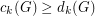for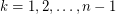.

(Reproduced from [M].)

Let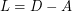be the Laplacian matrix of a graphof order. Let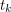be the-th largest eigenvalue of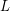(). For the purpose of this problem, we call the number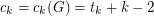the-th Laplacian degree of. In addition to that, let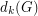be the-th largest (usual) degree in. It is known that every connected graph satisfiesfor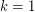[GM],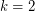[LP] and for[G].

Bibliography

[GM] R. Grone, R. Merris, The Laplacian spectrum of a graph II, SIAM J. Discrete Math.7 (1994) 221-229. MathSciNet

[LP] J.S. Li, Y.L. Pan, A note on the second largest eigenvalue of the Laplacian matrix of a graph, Linear Multilin. Algebra 48 (2000) 117-121. MathSciNet

*[G] J.-M. Guo, On the third largest Laplacian eigenvalue of a graph, Linear Multilin. Algebra 55 (2007) 93-102. MathSciNet

[M] B. Mohar, Problem of the Month

* indicates original appearance(s) of problem.